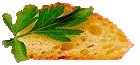Good Cooking since 1995

# Cooking Measures

How many times have you tried to remember how many tbsp. there are in a cup or how many ounces in a 1/2 gallon? What is a dram---The unit of volume is more correctly called a fluid dram and is specified to be 1/8 of a fluid oz.

 1 tsp. = 1/6 US fl oz 1 tbsp. = 3 tsp.s 1/4 cup = 4 tbsp.s 1/3 cup = 5 tbsp.s + 1 tsp. 1/2 cup = 8 tbsp. 2/3 cup = 1/2 cup + 2 tbsp. + 2 tsp. 1 cup = 16 tbsp. 1 pint = 2 cups 1 quart = 4 cups 1 gallon = 4 quarts 2 tbsp. = 1 ounce 1 cup = 48 tsp. 1 pint = 96 tsp. 1 pint = 32 tbsp. 1 quart = 64 tbsp. 1 gallon = 8 pints